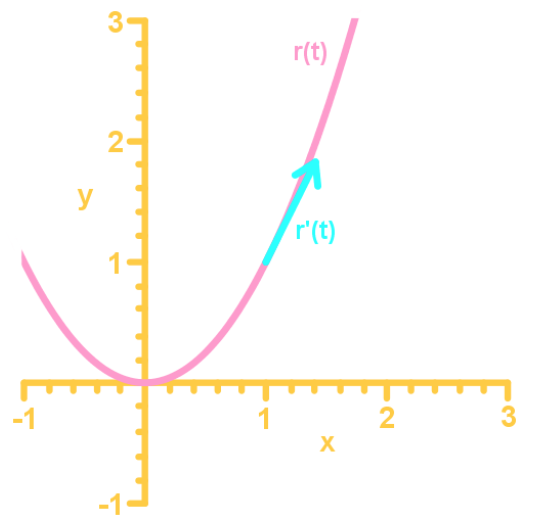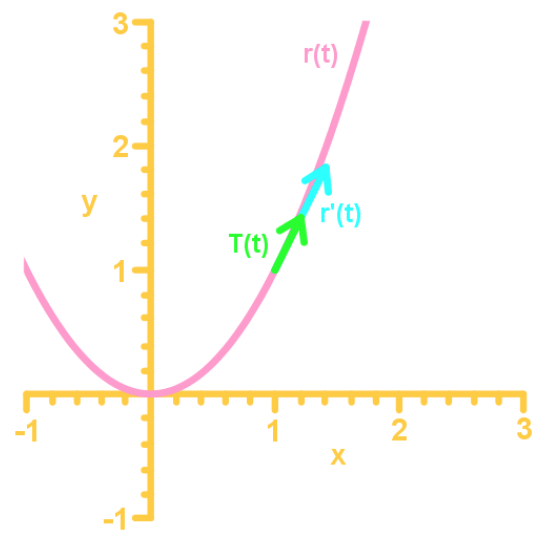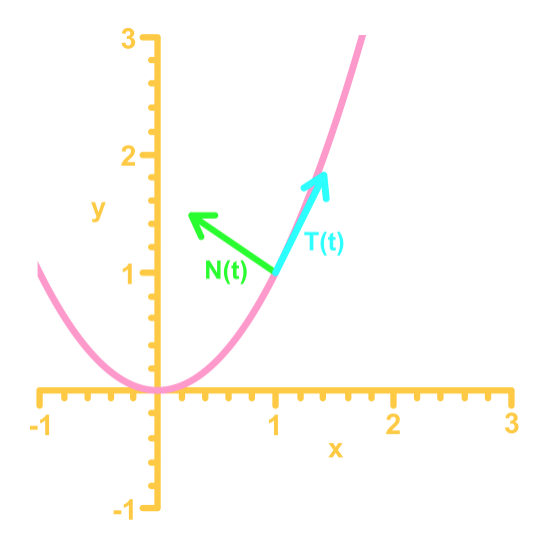# Tangent, normal, and binormal vectors

### Tangent, normal, and binormal vectors

#### Lessons

Notes:

Tangent & Unit Tangent Vectors
For a vector function $r(t)$, we call $r'(t)$ the tangent vector where $r'(t) \neq 0$. In a sense, $r'(t)$ is a tangent line to $r(t)$ at point $P$.The unit tangent vector is calculated by:

$T(t) = \frac{r'(t)}{||r'(t)||}$

Where $r'(t) \neq 0$. The unit tangent vector specifically has a length of one.Unit Normal Vector
A unit normal vector $N(t)$ is a vector that is orthogonal (or perpendicular) to the unit tangent vector $T(t)$ and to the vector $r(t)$.It is calculated by:

$N(t) = \frac{T'(t)}{||T'(t)||}$

Binormal Vector
A binormal vector $B(t)$ is a vector that is both orthogonal to the unit normal vector $N(t)$ and unit tangent vector $T(t)$. We can calculate $B(t)$ by taking the cross product of $N(t)$ and $T(t)$.

$B(t) = T(t) \times N(t)$

• Introduction
Tangent, Normal, & Binormal Vectors Overview:
a)
Tangent & Unit Tangent vectors
• $r(t) \to$ vector function
• $r'(t) \to$ tangent to the vector function
• $T(t) \to$ unit vector tangent to the vector function
• $T(t) = \frac{r'(t)}{||r'(t)||}$

b)
Unit Normal Vector
• $N(t) \to$orthogonal to $r'(t)$ and $T(t)$
• $N(t) = \frac{T'(t)}{||T'(t)||}$
• A unit vector (length of 1)

c)
Binormal Vector
• $B(t) \to$ orthogonal to both $T(t)$ and $N(t)$
• Calculated by taking the cross product $T(t) \times N(t)$

• 1.
Finding the Tangent Vector and Line
Find the tangent line to the vector function $r(t)= \lt t^3-2, 2+2t^2, t\gt$ at $t=1$.

• 2.
Find the tangent line to the vector function $r(t)= \lt\cos t, \sin t, \tan t\gt$ at $t= \pi$.

• 3.
Finding the Unit Tangent Vector Function
Find the unit tangent vector for the given vector function:

$r(t) = \lt e^{4t}, t^2 + 3, e^t \gt$

• 4.
Finding the Unit Normal Vector
Find the unit normal vector for the given vector function:

$r(t) = \lt \sin t, \cos t, 2t \gt$

• 5.
Find the unit normal vector for the given vector function:

$r(t) = \lt e^t \sin t, e^t \cos t, 1\gt$

• 6.
Finding the Binormal Vector
Find the binormal vector for the given vector function:

$r(t) = \lt \sin (t), \cos (t), t \gt$